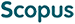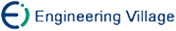宋传鸣, 王相海. 一种基于新量化方案的形状自适应整数小波编码算法[J]. 计算机研究与发展, 2006, 43(4): 695-701.
 引用本文: 宋传鸣, 王相海. 一种基于新量化方案的形状自适应整数小波编码算法[J]. 计算机研究与发展, 2006, 43(4): 695-701.Song Chuanming, Wang Xianghai. A Shape Adaptive Integer Wavelet Coding Algorithm Based on New Quantization Scheme[J]. Journal of Computer Research and Development, 2006, 43(4): 695-701.
 Citation: Song Chuanming, Wang Xianghai. A Shape Adaptive Integer Wavelet Coding Algorithm Based on New Quantization Scheme[J]. Journal of Computer Research and Development, 2006, 43(4): 695-701.## A Shape Adaptive Integer Wavelet Coding Algorithm Based on New Quantization Scheme

• 摘要: 首先提出了基于提升方案的形状自适应整数小波变换算法，然后对整数小波同第一代小波的变换系数分布特性的差异进行了详细的分析.针对整数小波变换系数幅值动态变化范围小，而传统EZW中阈值区间相对较大，不利于形成零树结构的不足，提出了基于奇数平方的阈值选取方法.并在此基础上，提出一种基于二分法的量化逼近方案，与传统的基于位平面的量化方法相比，该方案能用更少的比特来完全重构一个系数.最后，提出了基于整数小波变换和新的量化方案的形状自适应EZW编码算法.实验结果表明，阈值选取方法是合理的，而基于二分法的量化逼近方案是有效的.在相同码率下，与传统阈值选取和量化方案相比，该方法重构图像的峰值信噪比要高出0.5~2dB.

Abstract: A shape adaptive integer wavelet transform (IWT) algorithm based on lifting scheme is proposed. Through careful analysis of the difference of the coefficients' distribution characteristics between the integer wavelet and the first generation wavelet, it is concluded that the narrow dynamic range of IWT coefficients under a relatively wider threshold interval can result in much less zerotrees. Then a quantization threshold scheme based on an odd number square and a quantization strategy based on binary search are proposed. The scheme is able to use less bits than the scheme based on bit-plane to exactly reconstruct a coefficient. On this basis, a shape adaptive EZW based on IWT and the new binary quantization scheme is proposed. Simulation results prove the rationality of the threshold and the effectiveness of the binary quantization scheme. At the same decode bitrate, the proposed scheme can achieve S/N ratio, which is 0.5-2dB higher PSNR than the traditional threshold and bit-plane based quantization scheme./下载:  全尺寸图片 幻灯片
• 分享
• 用微信扫码二维码

分享至好友和朋友圈